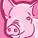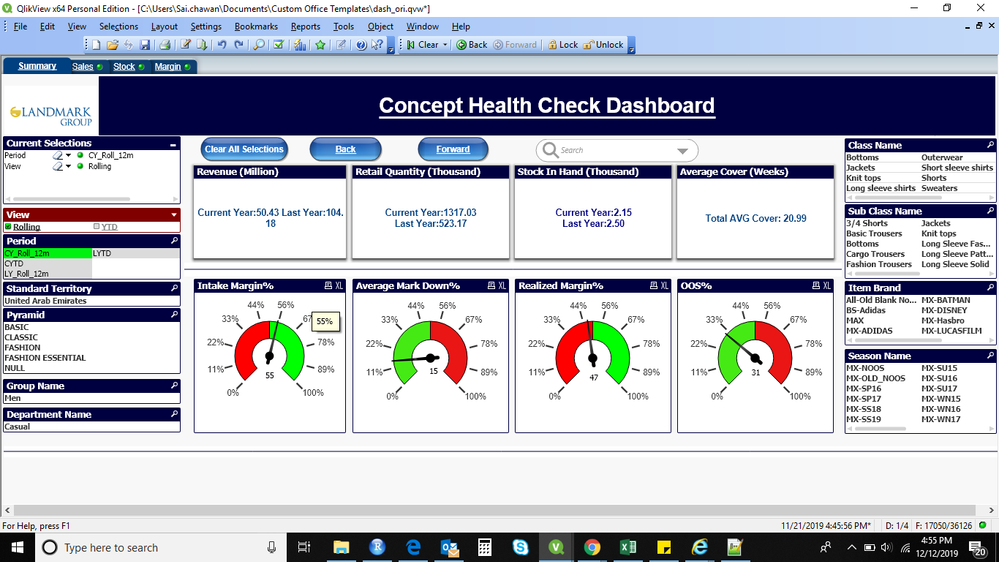# QlikView App Dev

Discussion Board for collaboration related to QlikView App Development.

cancel
Showing results for
Did you mean:Contributor III

## Rolling and ytd calculation

Hello,

I need to find rolling and YTD revenue. rolling month starts from september and ends on august. I have two years of data sep-2019 to aug-2018. i have a text object where current year and last revenue will be displayed. so, when i select rolling in the filter, in the text object revenue should displayed as currrent year rolling revenue and last year rolling revenue.

when i'm using the below code to get revenue, the current year revenue is coming up to be zero and last year revenue is getting switched with current year revenue. Attaching the screenshot of the dashboard for your reference.

='Current Year:'& Num(Sum({<[week] = {">=\$(=MakeDate(Year(Today()),9,1) )<=\$(=MakeDate(Year(Today())+1,8,30) )"}> } NET_SLS_AMT/1000000),'##.00')
& ' Last Year:'&Num(Sum({<[week] = {">=\$(=MakeDate(Year(Today())-1,9,1) )<=\$(=MakeDate(Year(Today()),8,30) )"}> } NET_SLS_AMT/1000000),'##.00')

And when i tried changing the code with

='Current Year:'& Num(Sum({<[week] = {">=\$(=MakeDate(Year(Today()-1),9,1) )<=\$(=MakeDate(Year(Today()),8,30) )"}> } NET_SLS_AMT/1000000),'##.00')
& ' Last Year:'&Num(Sum({<[week] = {">=\$(=MakeDate(Year(Today())-2,9,1) )<=\$(=MakeDate(Year(Today()-1),8,30) )"}> } NET_SLS_AMT/1000000),'##.00')

With the above formula, rolling current year and rolling last year values are coming out to be same.

PFA of sample Data and screenshot13 RepliesContributor III
Author

Sample dataCreator II

Hi,

Attached is the solution to your problem.

If your query is resolved then Mark it as Solution.Contributor III
Author

hey, thanks a ton for your solution. current year data is matching, but for the last the revnue is suming up for 2 years.Creator II

Basis the shared data,if you select Period as CY_Roll_12m then your current month value comes as 50.43 and Last year 53.75

If you select Period as LY_Roll_12m then your current month value comes as 53.75 and Last year 00.00Contributor III
Author

attahment of dashboard. after using  the above code

='Current Year:'& Num(Sum({<week1 = {">=\$(vMinDate)<=\$(vMaxdate)"}> } NET_SLS_AMT/1000000),'##.00')
& ' Last Year:'&num((Sum({<[week] = {">=\$(vRollingStart)<=\$(vRollingEnd)"},Period=> } NET_SLS_AMT/1000000)
-
Sum({<[week] = {">=\$(vRollingStart)<=\$(vRollingEnd)"},Period={'*YTD*'},Period= {'LYTD'}> } NET_SLS_AMT/1000000)),'##.00')Creator II

Are you using the same dataset? if not then given me all entire data.Contributor III
Author

PFA

dataset and qlickviewContributor III
Author

QVW fileCreator II

Here is the application with Solution. you did not add the variable.

If this resolved the accept this as solution.

Regards,

Imran K